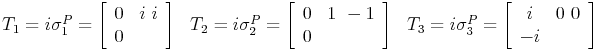2007-06-07 13:46:59 tex testing
My Tex system is pooched, so I'm just doing this code page to test rendering this lone image:$tex image$f(x) = \sqrt{1+x} \quad (x \ge -1 )
• Jeff (Thu, June 7th, 2007, 4:35pm UTC)
yea! working again.

• JEff (Tue, July 3rd, 2007, 3:14pm UTC)$tex image$
\begin{array}{ccc}
T_1 = i \sigma_{1}^{P} = \left[\begin{array}{cc}
0 & i\\
i & 0\end{array}\right] &
T_2 = i \sigma_{2}^{P}=\left[\begin{array}{cc}
0 & 1\\
-1 & 0\end{array}\right] &
T_3 = i \sigma_{3}^{P}=\left[\begin{array}{cc}
i & 0\\
0 & -i \end{array}\right]\end{array}

• Jeff (Wed, August 29th, 2007, 3:16am UTC)
I do, however, need to get rid of the hover text and make the images clickable to show the source…

• john Joseph Bachir (Sun, September 9th, 2007, 10:16pm UTC)
niiiiiice

• Jeff (Mon, September 10th, 2007, 12:29am UTC)
As John noted, the images are now clickable, revealing copyable LaTeX raw source. Clicking again on the raw source brings back the image. Some tiny design glitches with the image positioning in certain cases, but a big improvement from what I had.

Leave a comment